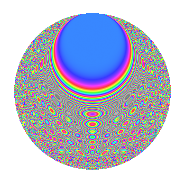# Properties

 Label 3150.2.bxLevel 3150 Weight 2 Character orbit bx Rep. character $$\chi_{3150}(629,\cdot)$$ Character field $$\Q(\zeta_{10})$$ Dimension 320 Sturm bound 1440

# Related objects

## Defining parameters

 Level: $$N$$ = $$3150 = 2 \cdot 3^{2} \cdot 5^{2} \cdot 7$$ Weight: $$k$$ = $$2$$ Character orbit: $$[\chi]$$ = 3150.bx (of order $$10$$ and degree $$4$$) Character conductor: $$\operatorname{cond}(\chi)$$ = $$525$$ Character field: $$\Q(\zeta_{10})$$ Sturm bound: $$1440$$

## Dimensions

The following table gives the dimensions of various subspaces of $$M_{2}(3150, [\chi])$$.

Total New Old
Modular forms 2944 320 2624
Cusp forms 2816 320 2496
Eisenstein series 128 0 128

## Trace form

 $$320q - 80q^{4} + O(q^{10})$$ $$320q - 80q^{4} - 80q^{16} - 40q^{22} + 20q^{28} + 24q^{46} - 8q^{49} - 80q^{64} + 60q^{70} - 16q^{79} + 40q^{88} - 104q^{91} + O(q^{100})$$

## Decomposition of $$S_{2}^{\mathrm{new}}(3150, [\chi])$$ into newform subspaces

The newforms in this space have not yet been added to the LMFDB.

## Decomposition of $$S_{2}^{\mathrm{old}}(3150, [\chi])$$ into lower level spaces

$$S_{2}^{\mathrm{old}}(3150, [\chi]) \cong$$ $$S_{2}^{\mathrm{new}}(525, [\chi])$$$$^{\oplus 4}$$$$\oplus$$$$S_{2}^{\mathrm{new}}(1050, [\chi])$$$$^{\oplus 2}$$$$\oplus$$$$S_{2}^{\mathrm{new}}(1575, [\chi])$$$$^{\oplus 2}$$

## Hecke Characteristic Polynomials

There are no characteristic polynomials of Hecke operators in the database